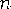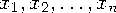Functions and CALL Routines

# RMS Function

Returns the root mean square of the nonmissing arguments.
 Category: Descriptive Statistics

## Syntax

 RMS(argument<,argument,...>)

### Arguments

argument

is a numeric constant, variable, or expression.

 Tip: The argument list can consist of a variable list, which is preceded by OF.

The root mean square is the square root of the arithmetic mean of the squares of the values. If all the arguments are missing values, then the result is a missing value. Otherwise, the result is the root mean square of the non-missing values.

Letbe the number of arguments with non-missing values, and letbe the values of those arguments. The root mean square isSAS Statements Results
`x1=rms(1,7);`
`5`
`x2=rms(.,1,5,11);`
`7`
`x3=rms(of x1-x2);`
`6.0827625303`Previous Page | Next Page | Top of Page